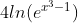## Natural Log

Determine the value of:Hint
The logarithm of $$x$$ to the Base $$b$$ is defined by
$$log_b(x)=c$$$where $$b^c=x$$ . Hint 2 Special definitions when $$b=e$$ or $$b=10$$ are: • ln $$x$$ → Base = $$e$$ • log $$x$$ → Base = 10 The logarithm of $$x$$ to the Base $$b$$ is defined by $$log_b(x)=c$$$
where $$b^c=x$$ . Special definitions when $$b=e$$ or $$b=10$$ are:
• ln $$x$$ → Base = $$e$$
• log $$x$$ → Base = 10

Analyzing the natural log section:
$$ln(e^{x^3-1})=ln_e(e^{x^3-1})=(x^3-1)$$$Since the original equation had a multiple of 4: $$4(x^3-1)=4x^3-4$$$
$$4x^3-4$$\$